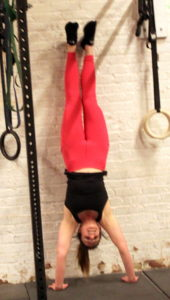## Thursday, May 2, 2019

### Thursday, May 2, 2019five cycles, as many repetitions as possible in three minutes; rest exactly one minute between cycles:
6x dumbbell power clean @ 50/35-lb. dbs
8x lateral burpee

### 1 Comment

1.–Scores–
Kate H.: 10 + 6x pc (20-lb. dbs)
Borja G.: 15 + 1x burpee (40-lb. dbs)
Al C.: 11 + 2x burpee
Aaron B.: 11 rounds (35-lb. dbs)
Anna G.: 11 + 5x pc (25-lb. dbs)
Rami B.: 10 + 5x burpee (30-lb. dbs)
Will O.: 12 + 1x burpee (40-lb. dbs)
Kevin K.: 11 rounds (35-lb. dbs; sub: no pushup burpee)
David C.: 11 + 6x pc (35-lb. dbs)
Chase H.: 12 rounds
Hari P.: 13 rounds (40-lb. dbs)
Tommy W.: 11 + 3x pc (30-lb. dbs)
Charles B.: 14 + 3x box jump (225-lbs.; sub: 6x deadlift + 6x true strict bodyrow + 12x box jump, 12″)
Walt C.: 11 + 4x pc (35-lb. dbs)
Jason S.: 10 + 2x pc (30-lb. dbs)
Drew P.: 18 + 6x dl (75-lbs.; sub: kb deadlift)
Ralph B.: 12 + 7x burpee (35-lb. dbs; sub: hang power clean)
Gabe P.: 14 rounds
JP S.: 14 + 1x pc (35-lb. dbs; sub: lateral burpee step-over)
Andrew B.: 11 + 4x burpee (30-lb. dbs)
Bill M.: 12 + 4x burpee (75-lbs.; sub: kbn deadlift)
Cristian D.: 12 rounds (30-lb. dbs; sub: hang power clean)
Diana D.: 9 + 6x pc (15-lb. dbs)
Adrienne V.: 11 + 2x pc (25-lb. dbs)
Andrew Z.: 14 + 1x pc (40-lb. dbs)
Stephen K.: 11 rounds (40-lb. dbs)
Simon B.: 12 + 1x burpee (25-lb. dbs; sub: lateral burpee step over)
Bill G.: 12 + 4x burpee (sub: hang power clean)
Anna Go.: (30-lb. dbs; sub: step-over burpee)
Dan C.: 10 + 2x pc (35-lb. dbs)
Tarek K.: 10 + 6x pc (30-lb. dbs)
Eddie: 11 + 2x pc (40-lb. dbs)
Charlie S.: 11 + 1x burpee (40-lb. dbs)
Josh M.: 12 + 3x pc (45-lb. dbs)
Zach D.: 12 + 3 bj (70-lb. db; sub: single-arm pc, box jump + lateral jump)
Anna Go.: 9 + 4x burpee (30-lb. dbs; sub: step-over burpee)
Alli P.: 14 + 5x pc (30-lb. dbs)
RJ A.: 11 + 3x pc (30-lb. dbs)
John S.: 10 rounds (30-lb. dbs)
Jarod F.: 7 + 6x pc
Seth B.: 10 + 3x pc (25-lb. dbs)
Colin So.: 10 + 1x burpee (20-lb. dbs)
Aron S.: 7 rounds (25-lb. dbs)
Matt K.: 11 rounds (30-lb. dbs)
Carl L.: 10 + 3x burpee (40-lb. dbs)
Shari F.: 10 rounds (10-lb. dbs; sub: step-up, 20″)
Terry A.: 5 rounds (10-lb. dbs; sub: burpee)
Yao Z.: 12 rounds + 2x pc (20-lb. dbs; sub: step-over burpee)
Tom G.: 9 + 3x burpee (15-lb. dbs; sub: step-over burpee)
Mark Co.: 10 + 2x pc
Jin D.: 13 + 2x pc